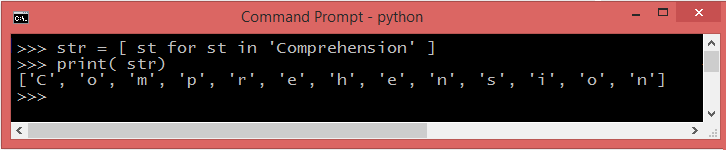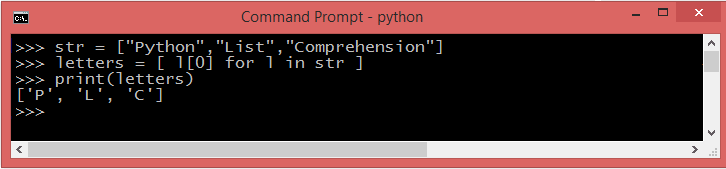Net-informations.com

# Python list comprehension

List comprehension is one of the most most distinctive feature in Python , which you can use to create powerful functionality within a single line of code . It is an elegant way to define and create lists based on existing lists. Essentially, it is Python's way of implementing a well-known notation for sets as used by mathematicians.As list comprehensions return lists, they consist of brackets containing the expression followed by a for clause, which is executed for each element along with the for loop to iterate over each element. The expressions can be anything, meaning you can put in all kinds of objects in lists.

#### Syntax

This is equivalent to Python for loop:

#### example

The following code will create List using List Comprehension.

## Python list comprehension exercises

Create a list of squares using List Comprehension.

The above code is equivalent to:

### Iterating through a string using List Comprehension## Using Conditionals in List Comprehension

### if statement in List comprehension

List comprehension is an example of the language's support for functional programming concepts . You can modify the existing list using conditional statements in List comprehension .

The above code is equivalent to:

### if..else ststement in List comprehension

Here we have a list containing numbers 1 to 10. If the item in list less than or equal to 5 then the number is added to 10 and if the number is greater than 5 then the item is added to 100.

### List comprehension with multiple if's (nested if)

Following Python script produces the integers between 11 and 19, inclusive.

The above code is equivalent to:

## Nested List Comprehensions

Consider the following example of a 3x4 matrix implemented as a list of 3 lists of length 4 as "Name", "Marks" and "Grade".

You can use the following list comprehension will transpose rows and columns:

#### output

The above code is equivalent to:

### First letter of each word using list comprehension## Python list comprehension in functions

Python's list comprehension is an example of the language's support for functional programming concepts . Letss see how you can use list comprehension in functions.

## How to read a file line-by-line using List Comprehension

Create a text file and add some content and save its as "testfile.txt".

## List Comprehensions Vs Map and Lambda functions

List comprehension is a complete substitute for the lambda function as well as the functions map(), filter() and reduce() . Unless you're applying a single-argument function, list comprehensions are clearer than the map built-in function for simple cases.

### Using List Comprehension

net-informations.com (C) 2022    Founded by raps mk